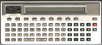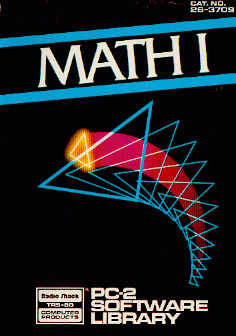##### TRS-80 PC-2 (and Sharp PC-1500) : Math Pak I

Radio Shack TRS-80 PC-2 Math Pak I software(*).PC-2 Math Pak I: © 1982 Tandy Corporation. All rights reserved. "Math Pak I" consists of 5 programs: 1. TRIANGLE - Solution of triangles. 2. COMPLEX - Complex arithmetic. 3. VECTOR - Vector arithmetic. 4. ADVMATH - Advanced trigonometry and exponentials. 5. PCALC - Polynomial math and calculus.